Prof. Robert B. Laughlin, Department of Physics, Stanford University

Binary Pulsars

Mike McCourt December 10, 2007

(Submitted as coursework for Physics 210, Stanford University, Autumn 2007)Fig. 1 Hubble Space Telescope image of the crab nebula, the center of which contains a pulsar. (Courtesy of STScl/NASA).

Introduction

This paper is about binary pulsars, or pular stars with binary companions. Pulsars are astronomical objects which emit light at extraordinarily regular intervals, with accuracies approaching those of modern atomic clocks. Pulsars can therefore be used to make very precise timing measurements and, if the binary companion to a pulsar is a relativistic object (such as a neutron star or black hole), the binary system can offer a rare test of Einstein's theory of general relativity in the strong field limit. The first binary pulsar was discovered by Joseph Taylor and Russell Hulse in 1974 during a search for new pulsars at the Arecibo telescope. The pulsar is named PSR 1913+16, and is in a highly elliptical orbit around its companion neutron star with a period (or year) of around eight hours. Measurements of the timing signals can be fit to a relatively simple 10-parameter model incorporating variables from the pular timing, the Keplerian orbits and relativistic corrections for periastron advance and gravitational redshift. The fit to these last two corrections provides a test of general relativity in a gravitational field orders of magnitude stronger than that found in our solar system, but the most important result to be gleaned from this pulsar is the fact that a good fit to the data cannot be obtained without the inclusion of a term for energy loss due to gravitational radiation. This provided the first evidence for the existence of gravitational waves, which are predicted by general relativity but which have never been directly observed. Hulse and Taylor were awarded the 1993 Nobel Prize for their discovery.

In the following section, I will summarize some basic results about binary systems, and in the third section, I will outline some of the relativistic corrections to the Keplerian orbits presented in second section. Finally, in fourth section, I will apply these relations to the specific pulsar PSR 1913+16 mentioned above.

Binary Systems

A binary system consists of two gravitationally bound objects in orbit about a common center of mass. Observations of the dynamics of binary systems are the primary source of information about stellar masses. Newton's law of gravitation suffices to describe most binary systems, and I will summarize the description in this section. PSR 1913+13 is significant because its dynamics require a relativistic treatment of gravity and I will outline the most important corrections in the third section.

Binary systems are classified as either visual or spectroscopic, according to how we are able to observe them. Visual binaries are stars which can be seen to be double; their separations can be directly measured, but they have large separations and long periods and are therefore not relevant as tests of relativistic gravity. A spectroscopic binary system is one in which the individual stars cannot be separately resolved, but in which the orbital motion can be inferred from a periodic doppler shift in the spectrum of the brighter star.

Binary stars obey Kepler's third law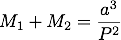where a is the semimajor axis of the orbit and P is its perion. These stars move in elliptical orbits about their common center of mass, but we can also describe the system in terms of a relative orbit, in which one star is considered fixed and the other orbits it. This orbit is also an ellipse and also obeys Kepler's laws. Finally, when we observe a binary system, we see this ellipse projectd onto the sky, at some inclination angle i. In a visual binary system, the mass of the whole system can be inferred from Kepler's third law, while for a spectroscopic binary, only the radial velocity, and not the separation is known, so the total mass of the system cannot be determined. The quantity M23 sin3i / (M1 + M2) can be calculated, however.

Relativistic Corrections

The dynamics of most binary stars can be determined from Kepler's third law, but compact objects may not be adequately described by Newtonian gravity. Observations of the binary pulsar PSR 1923+16 indicate that its orbit about its companion precesses considerably, in violation of Kepler's first law, and also indicates that the pulsar is slowly spiraling into its companion, seemingly in violation of the law of conservation of energy. A relativistic description of this pulsar's orbit is required to account for these effects and I will attempt to describe them in this section.

I will introduce the concepts of gravitational redshift, precession of the periastron and energy loss due to gravitational radiaion in this section. To simplify my descriptions of the first two effects, I will demonstrate the results only in the Schwarzschild geometry. Furthermore, to simplfy the equations, I will use geometrized units, in which G = c = 1. This unit system requires measuring time in centimeters, which is very common in relativity and which has the same simple interpretation as the "light-year" unit of distance. This unit system also requires measuring mass in units of centimeters, which may be less familiar, but which follows from the simple formula: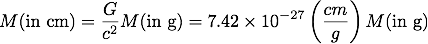The measurements has the rough interpretation that, when the mass and radius are comparable in length, the effects of general relativity become important. We can check this against the values for Earth to find that R&oplus &asymp 600,000,000 cm, while, as expected, the mass is much smaller: M&oplus &asymp 0.44 cm.

Next, I need to introduce the Schwarzchild metric,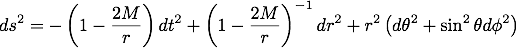which specifies the line element in the geometry near a spherically symmetric point-mass distribution and can be interpreted as the matrix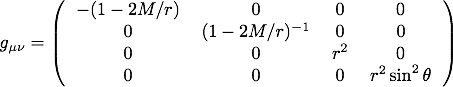Precession of the Periastron

The first relativistc correction to add to our model of binary systems is the precession of the pulsar's periastron in its binary orbit. This is identical in nature to the famous precession of Mercury's perihelion in our solar system, which provded a critical early test of general relativity, but the effect can be much more pronounced. The periastron of binary pulsar PSR 1913+6, for example, advances as much in a single day as Mercury's does in a century.

It is generally very cumbersome to determine orbits in general relativity; fortunately, the study of orbits in the Schwarzchild geometry is considerably simplified by the symmetries of the metric. The metric is invariant under time translation, leading to the conservation of energy, and it is also invariant under angular rotations, leading to the conservation of angular momentum. These conserved quantites take the forms: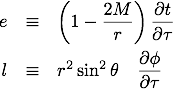where t is the Schwarzchild time and &tau is the proper time elapsed along the orbit. Just as in the Newtonian theory of gravity, conservation of angular momentum requires that orbits in a Schwarzchild geometry lie "in a plane." From now on, therefore, I will work only in the equatorial plane &theta = &pi/2.

Finally, we can use the normalization of the four-velocity,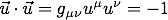which provides enough information to solve for the orbits. Using this normalization while replacing the angular and time derivatives with the above conserved quantities, we have the equation of motion: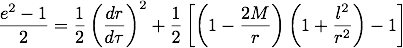We can use the following definitions,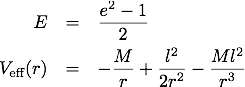to rewrite the equation of motion in a more familiar form, directly analogous to Newtonian orbits: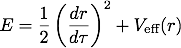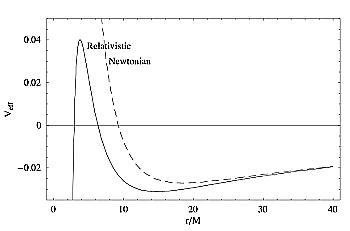Fig. 2: Plot of effective 2-body potential Veff for the binary pulsar showing relativistic softening of the centrifugal barrier.

Notice that the effective potential Veff(r) is idential to the Newtonain one, with an extra term proportional to r -3. I've plotted a comparison of the two effective potentials in Fig. 2. The fact that orbits are closed in Newtonian gravity is a very sensitive consequence of the r -2 force law and it should be clear that the orbits will not usually close in general relativity. It is straightforward to divide the two conserved quantities to obtain a formula for (&part r / &part &phi), which can then be integrated over one orbit to obtain the value for the precession. The precession turns out to be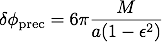where a is the semimajor axis of the orbit and &epsilon is its eccentricity. A more thorough calculation of the precession (quoted in Ref ) gives the rate of precession: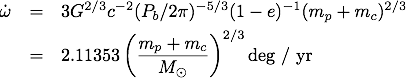To summarize, we have taken the laws of conservation of energy and of angular momentum, along with the normalization condition for vectors in general relativity to obtain an equation of motion for a particle in a Schwarchild geometry, without having to solve the dreadead geodesic equation. We found that, just as in the Newtonian theory, the problem can be reduced to motion in a single dimension with the effective potential Veff(r). The Schwarzchild effective potential differs from the Newtonian one by the addition of a term proportional to r -3, meaning that the familiar Keplerian orbits of Newtonian theory are actually impossible around a Schwarzchild mass and that all orbits must precess somewhat. This precession is typically very small, however, and the Keplerian result is recovered in the weak field limit.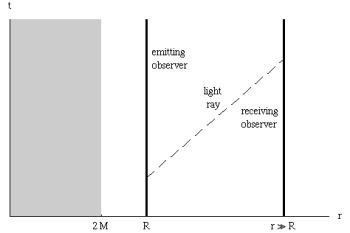Fig. 3: Illustration of time-dilation effects near a pulsar surface.

Gravitational Redshift

Consider the pulsar stationed at some radius R from its Schwarzchild binary companion. If the pulsar is emitting light at a frequency &omega*, astronomers on Earth will recieve the signals at a lower frequency, &omega&infin, due to the gravitational redshift effect.

This gravitational time dilation can be read almost directly from the Schwarzchild metric; again, the normalization condition means that the pulsar must satisfy gtt (R) [ut (R)]2 = -1, or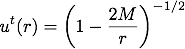Observers on Earth must of course satisfy the same condition, but their r coordinate is so large that ut &asymp 1. We therefore obtain the simple relation illustrated in Fig. 3: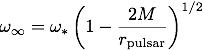If the pulsar is in an elliptical orbit about its binary companion, the value of rpulsar will change throughout the orbit, and astronomers on Earth can measure this change and infer the period and semimajor axis of the pulsar orbit. In reality, of course, there are complicating effects such as the relativistic Doppler shift, as discussed in second section. Again, a more thorough calculation taken from Ref  gives: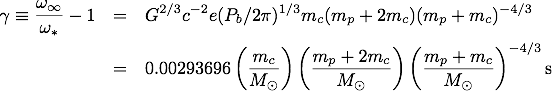Gravitational waves are fluctuations in the curvature of spacetime in the absence of nearby sources of stress-energy. In this section, I will describe these waves and will present the solution for gravitational waves produced by a binary system of point masses. As usual, I will work only in the weak field limit to simplify the calculation. Specifically, I will assume the following form of the metric: g&mu&nu = &eta&mu&nu + h&mu&nu, where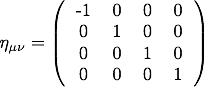is simply the flat space-time metric of special relativity, and |h&mu&nu| << 1 are small corrections to |&eta&mu&nu. We want to solve the equations of motion for h&mu&nu to obtain a gravitational wave solution.

If we choose a fixed coordinate system relative to the Minkowski background, we can decompose h&mu&nu into a traceless tensor s&mu&nu, a three vector wi and a scalar &Psi. We therefore rewrite h&mu&nu as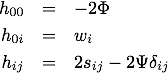where &Psi encodes the trace of h&mu&nu and s&mu&nu is traceless. The solution of the Einstein equations then gives: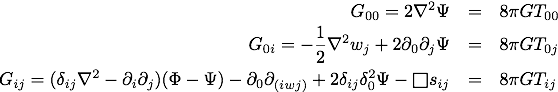In the absence of sources of stress-energy, T&mu&nu = 0, and the 00 equation becomes &nabla2&Psi = 0, which implies &Psi = 0 for reasonable boundary conditions. Plugging this into the 0j equation similarly shows that wj = 0. Putting these two results into the trace part of the ij equation then shows that &Phi = 0, so we are left with only the trace-free part of the ij equation, which reads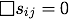Finally, we have rewritten h&mu&nu in a traceless form, as follows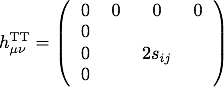which satifsies the wave equation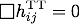and has the solutions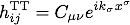The solutions are only nontrivial if k&sigmak&sigma = 0, which requires that the waves travel at the speed of light. If we assume that the wave is simply a plave wave of fixed frequency and orient our coordinates such that the wave travels only along the x3 coordinate, then k&mu = (&omega,0,0,&omega), and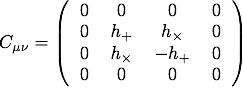These are the "plus" and "cross" polarizations of gravitational plane waves; just like in electricity and magnetism, they can be combined to produce right and left handed circular polarizations: hR,L = h+ ± i h×.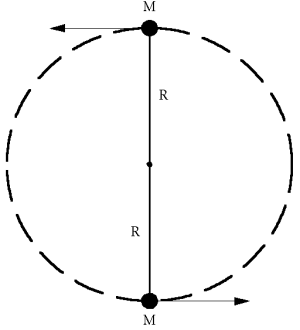Fig. 4: Idealized geometry for computing gravitation radiation.

So we have found a particular kind of metric perturbation which, at least in the weak field limit, behaves like a gravitational wave. Like electromagnetic waves, these waves travel at the speed of light and can have either linear or circular polarizations. I should also mention that the time dependent parts of the solutions we just found are essentially Fourier modes and can be combined to make more general types of waves.

I would now like to introduce the generation of these waves by a binary system. I will assume (cf. Fig. 4) that two stars of equal mass M are in circular orbits about their common center of mass, with orbital frequency &Omega. Furthermore, I will impose the weak field condition, so that the orbits can be approximated as Keplerian. The T&mu&nu tensor is now nonzero and the equation for the metric perturbations is much more difficult to solve. The solution is found in Ref and is as follows:

h+ = - 8 (M &Omega2 R2 cos 2&Omega tr) / r

and that

h+ = - 8 (M &Omega2 R2 sin 2&Omega tr) / r,

where tr is the retarded time, t - |r|, simply the time at which the waves arriving at time t were produced. A more thorough calculation referenced in Ref  shows that the energy loss due to this gravitational radiation will result in a decrease in the orbital period: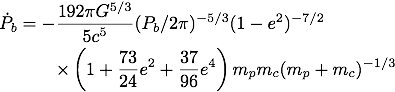Keplerian Parameters
Right ascension (1950) &alpha = 19h 13m 12.474 ± 0.004s
Declination (1950) &delta = 16° 01'08."02 ± 0".06
Orbital eccentricity e = 0.617155 ± 0.000007
Binary orbit period Pb = 27906.98172 ± 0.00005 s
Projected semimajor axis a1 sin i = 2.3424 ± 0.0007 light s
Sine of inclination angle sin i = 0.81 ± 0.16
Relativistic Corrections
Pulsar period P = 0.059029995269 ± 2 s
Derivative of pulsar period &partt P = (8.64 ± 0.02) × 10-18
Derivative of orbit period &partt Pb = (-3.2 ± 0.6) × 10-22
Rate of advance of periastron &partt &omega = 4.226 ± 0.002 ° yr-1
Transverse doppler and gravitational redshift &gamma = 0.0047 ± 0.0007 s
Table 1: Parameters and fits to timing data for PSR 1913+6.

PSR 1913+16

An important feature of equations 3.5 and 3.7b is that, if d&omega / dt and &gamma are measured, along with the Keplerian orbit parameters, you can solve simultaneously for mp, the mass of the pulsar and mc, the mass of the companion. The results give

mp = 1.42 ± 0.6 Msun
mc = 1.41 ± 0.6 Msun

This is the most precise measurement of the mass of a radio pulsar to date, providing an example of how general relativity can be used as a tool for precision cosmology.

The masses obtained for the binary and for its companion can then be plugged into the relation 3.9 to obtain an estimate for dP/dt, which, in the case of PSR 1913+16, this agrees to within 4% of the measured value. This measurement provides the best available evidense for the existence of gravitational radiation.

Armed with the masses of the binary stars, we can use Kepler's law to work out the rest of the orbital parameters: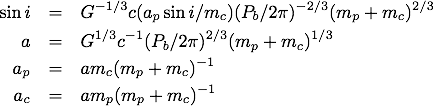All of these results are summarized in Table 1, taken from Ref .

© 2007 Mike McCourt. The author grants permission to copy, distribute and display this work in unaltered form, with attributation to the author, for noncommercial purposes only. All other rights, including commercial rights, are reserved to the author.

References

 J. Weisberg and J. Taylor, "The Relativistic Binary Pulsar B1913+16: Thirty Years of Observations and Analysis", astro-ph/0407149 (2004).

 L. D. Landau and E. M. Lifshitz, The Classical Theory of Fields (Pergamon, 1975), p. 356.

 S. M. Carroll, Spacetime and Geometry: An Introduction to General Relativity (Addison Wesley, 2004), p. 2742.